Name:    Geometry Chapter 11 Practice Test

Multiple Choice
Identify the choice that best completes the statement or answers the question.

1.

A rectangle has length l and width w. An expression for its area is _____.
 a. 2(l + w) c. lw b.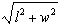d.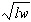2.

What is the circumference of a circle with a radius of 4.2 inches?
 a. 13.2 in. b. 6.6 in. c. 8.4 in. d. 55.4 in. e. 26.4 in.

3.

What is the length of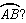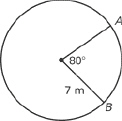4.

If both the base and height of a triangle are made nine times as long, how many times the area of the original figure is the area of the new figure?
 a. nine times c. eighty-one times b. ninety times d. thirty-six times

5.

The figure below is made up of two squares with the areas shown. What is the length of x?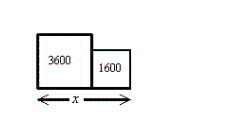a. 240 c. 650 b. 100 d. 2600

6.

Find the area of the parallelogram.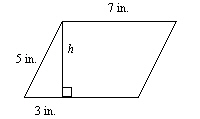a. 35 inc. 21 inb. 14 ind. 28 in7.

A circle has a circumference of 37 meters. Find its diameter.
 a. 11.78 m c. 5.89 m b. 9.25 m d. 18.5 m

8.

Find the area of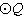in terms of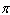.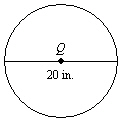a. 400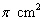c. 200b. 100d. 1009.

An expression for the area of the trapezoid is _____.a.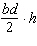c.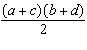b.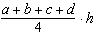d.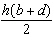10.

Find the area of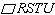.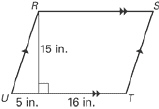a. 253 in.2 b. 332 in.2 c. 277.5 in.2 d. 240 in.2 e. 315 in.2